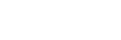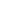Go is a static, compiled language created by Google. Go has advanced memory safety, garbage collection, structural typing, and CSP-style concurrency. Presently, Go is the favorite language of young developers.

## Popular New Releases in Go

 kubernetes Kubernetes v1.24.0-rc.0 moby v20.10.12 hugo v0.97.3 gin Release v1.7.7 frp v0.41.0
 kubernetesKubernetes v1.24.0-rc.0 mobyv20.10.12 hugov0.97.3 ginRelease v1.7.7 frpv0.41.0

1

321 Libraries

21651

2

316 Libraries

0

3

268 Libraries

183422

4

235 Libraries

180804

5

233 Libraries

18842

6

212 Libraries

7560

7

198 Libraries

2566

8

195 Libraries

0

9

188 Libraries

1559

10

159 Libraries

13

1

321 Libraries

21651

2

316 Libraries

0

3

268 Libraries

183422

4

235 Libraries

180804

5

233 Libraries

18842

6

212 Libraries

7560

7

198 Libraries

2566

8

195 Libraries

0

9

188 Libraries

1559

10

159 Libraries

13

## Trending Discussions on Go

QUESTION

Why is Rust NLL not working for multiple borrows in the same statement?

First, I tried something like this:

``````1let mut vec = vec!;
2vec.rotate_right(vec.len());
3``````

It can't be compiled because:

error[E0502]: cannot borrow `vec` as immutable because it is also borrowed as mutable

I thought that the Rust borrow checker could be smarter than this, so I found something called NLL, and it should solve this problem.

I tried the sample:

``````1let mut vec = vec!;
2vec.rotate_right(vec.len());
3let mut vec = vec!;
4vec.resize(vec.len(), 0);
5``````

It could work, but why is it not working with `rotate_right`? Both of them take a `&mut self`. What's going on?

It is definitely an interesting one.

They are similar - but not quite the same. `resize()` is a member of `Vec`. `rotate_right()`, on the other hand, is a method of slices.

`Vec<T>` derefs to `[T]`, so most of the time this does not matter. But actually, while this call:``````1let mut vec = vec!;
2vec.rotate_right(vec.len());
3let mut vec = vec!;
4vec.resize(vec.len(), 0);
5vec.resize(vec.len(), 0);
6``````

Desugars to something like:``````1let mut vec = vec!;
2vec.rotate_right(vec.len());
3let mut vec = vec!;
4vec.resize(vec.len(), 0);
5vec.resize(vec.len(), 0);
6&lt;Vec&lt;i32&gt;&gt;::resize(&amp;mut vec, &lt;Vec&lt;i32&gt;&gt;::len(&amp;vec), 0);
7``````

This call:``````1let mut vec = vec!;
2vec.rotate_right(vec.len());
3let mut vec = vec!;
4vec.resize(vec.len(), 0);
5vec.resize(vec.len(), 0);
6&lt;Vec&lt;i32&gt;&gt;::resize(&amp;mut vec, &lt;Vec&lt;i32&gt;&gt;::len(&amp;vec), 0);
7vec.rotate_right(vec.len());
8``````

Is more like:``````1let mut vec = vec!;
2vec.rotate_right(vec.len());
3let mut vec = vec!;
4vec.resize(vec.len(), 0);
5vec.resize(vec.len(), 0);
6&lt;Vec&lt;i32&gt;&gt;::resize(&amp;mut vec, &lt;Vec&lt;i32&gt;&gt;::len(&amp;vec), 0);
7vec.rotate_right(vec.len());
8&lt;[i32]&gt;::rotate_right(
9    &lt;Vec&lt;i32&gt; as DerefMut&gt;::deref_mut(&amp;mut vec),
10    &lt;Vec&lt;i32&gt;&gt;::len(&amp;vec),
11);
12``````

But in what order?

This is the MIR for `rotate_right()` (simplified a lot):``````1let mut vec = vec!;
2vec.rotate_right(vec.len());
3let mut vec = vec!;
4vec.resize(vec.len(), 0);
5vec.resize(vec.len(), 0);
6&lt;Vec&lt;i32&gt;&gt;::resize(&amp;mut vec, &lt;Vec&lt;i32&gt;&gt;::len(&amp;vec), 0);
7vec.rotate_right(vec.len());
8&lt;[i32]&gt;::rotate_right(
9    &lt;Vec&lt;i32&gt; as DerefMut&gt;::deref_mut(&amp;mut vec),
10    &lt;Vec&lt;i32&gt;&gt;::len(&amp;vec),
11);
12fn foo() -&gt; () {
13    _4 = &lt;Vec&lt;i32&gt; as DerefMut&gt;::deref_mut(move _5);
14    _6 = Vec::&lt;i32&gt;::len(move _7);
15    _2 = core::slice::&lt;impl [i32]&gt;::rotate_right(move _3, move _6);
16}
17``````

And this is the MIR for `resize()` (again, simplified a lot):``````1let mut vec = vec!;
2vec.rotate_right(vec.len());
3let mut vec = vec!;
4vec.resize(vec.len(), 0);
5vec.resize(vec.len(), 0);
6&lt;Vec&lt;i32&gt;&gt;::resize(&amp;mut vec, &lt;Vec&lt;i32&gt;&gt;::len(&amp;vec), 0);
7vec.rotate_right(vec.len());
8&lt;[i32]&gt;::rotate_right(
9    &lt;Vec&lt;i32&gt; as DerefMut&gt;::deref_mut(&amp;mut vec),
10    &lt;Vec&lt;i32&gt;&gt;::len(&amp;vec),
11);
12fn foo() -&gt; () {
13    _4 = &lt;Vec&lt;i32&gt; as DerefMut&gt;::deref_mut(move _5);
14    _6 = Vec::&lt;i32&gt;::len(move _7);
15    _2 = core::slice::&lt;impl [i32]&gt;::rotate_right(move _3, move _6);
16}
17fn foo() -&gt; () {
18    _4 = Vec::&lt;i32&gt;::len(move _5);
19    _2 = Vec::&lt;i32&gt;::resize(move _3, move _4, const 0_i32);
20}
21``````

In the `resize()` example, we first call `Vec::len()` with a reference to `vec`. This returns `usize`. Then we call `Vec::resize()`, when we have no outstanding references to `vec`, so mutably borrowing it is fine!

However, with `rotate_right()`, first we call `<Vec<i32> as DerefMut>::deref_mut(&mut vec)`. This returns `&mut [i32]`, with its lifetime tied to `vec`. That is, as long as this reference (mutable reference!) is alive, we are not allowed to use have any other reference to `vec`. But then we try to borrow `vec` in order to pass the (shared, but it doesn't matter) reference to `Vec::len()`, while we still need to use the mutable reference from `deref_mut()` later, in the call to `<[i32]>::rotate_right()`! This is an error.

This is because Rust defines an evaluation order for operands:

Expressions taking multiple operands are evaluated left to right as written in the source code.

Because `vec.resize()` is actually `(&mut *vec).rotate_right()`, we first evaluate the dereference+reference, then the arguments:``````1let mut vec = vec!;
2vec.rotate_right(vec.len());
3let mut vec = vec!;
4vec.resize(vec.len(), 0);
5vec.resize(vec.len(), 0);
6&lt;Vec&lt;i32&gt;&gt;::resize(&amp;mut vec, &lt;Vec&lt;i32&gt;&gt;::len(&amp;vec), 0);
7vec.rotate_right(vec.len());
8&lt;[i32]&gt;::rotate_right(
9    &lt;Vec&lt;i32&gt; as DerefMut&gt;::deref_mut(&amp;mut vec),
10    &lt;Vec&lt;i32&gt;&gt;::len(&amp;vec),
11);
12fn foo() -&gt; () {
13    _4 = &lt;Vec&lt;i32&gt; as DerefMut&gt;::deref_mut(move _5);
14    _6 = Vec::&lt;i32&gt;::len(move _7);
15    _2 = core::slice::&lt;impl [i32]&gt;::rotate_right(move _3, move _6);
16}
17fn foo() -&gt; () {
18    _4 = Vec::&lt;i32&gt;::len(move _5);
19    _2 = Vec::&lt;i32&gt;::resize(move _3, move _4, const 0_i32);
20}
21let dereferenced_vec = &amp;mut *vec;
22let len = vec.len();
23dereferencec_vec.rotate_right(len);
24``````

Which is obviously a violation of the borrow rules.

On the other hand, `vec.resize(vec.len())` has no work to do on the callee (`vec`), and so we first evaluate `vec.len()`, and then the call itself.

Solving this is as easy as extracting the `vec.len()` to a new line (new statement, to be precise), and the compiler also suggests that.

Community Discussions contain sources that include Stack Exchange Network

QUESTION

Why is Rust NLL not working for multiple borrows in the same statement?

First, I tried something like this:

``````1let mut vec = vec!;
2vec.rotate_right(vec.len());
3``````

It can't be compiled because:

error[E0502]: cannot borrow `vec` as immutable because it is also borrowed as mutable

I thought that the Rust borrow checker could be smarter than this, so I found something called NLL, and it should solve this problem.

I tried the sample:

``````1let mut vec = vec!;
2vec.rotate_right(vec.len());
3let mut vec = vec!;
4vec.resize(vec.len(), 0);
5``````

It could work, but why is it not working with `rotate_right`? Both of them take a `&mut self`. What's going on?

It is definitely an interesting one.

They are similar - but not quite the same. `resize()` is a member of `Vec`. `rotate_right()`, on the other hand, is a method of slices.

`Vec<T>` derefs to `[T]`, so most of the time this does not matter. But actually, while this call:``````1let mut vec = vec!;
2vec.rotate_right(vec.len());
3let mut vec = vec!;
4vec.resize(vec.len(), 0);
5vec.resize(vec.len(), 0);
6``````

Desugars to something like:``````1let mut vec = vec!;
2vec.rotate_right(vec.len());
3let mut vec = vec!;
4vec.resize(vec.len(), 0);
5vec.resize(vec.len(), 0);
6&lt;Vec&lt;i32&gt;&gt;::resize(&amp;mut vec, &lt;Vec&lt;i32&gt;&gt;::len(&amp;vec), 0);
7``````

This call:``````1let mut vec = vec!;
2vec.rotate_right(vec.len());
3let mut vec = vec!;
4vec.resize(vec.len(), 0);
5vec.resize(vec.len(), 0);
6&lt;Vec&lt;i32&gt;&gt;::resize(&amp;mut vec, &lt;Vec&lt;i32&gt;&gt;::len(&amp;vec), 0);
7vec.rotate_right(vec.len());
8``````

Is more like:``````1let mut vec = vec!;
2vec.rotate_right(vec.len());
3let mut vec = vec!;
4vec.resize(vec.len(), 0);
5vec.resize(vec.len(), 0);
6&lt;Vec&lt;i32&gt;&gt;::resize(&amp;mut vec, &lt;Vec&lt;i32&gt;&gt;::len(&amp;vec), 0);
7vec.rotate_right(vec.len());
8&lt;[i32]&gt;::rotate_right(
9    &lt;Vec&lt;i32&gt; as DerefMut&gt;::deref_mut(&amp;mut vec),
10    &lt;Vec&lt;i32&gt;&gt;::len(&amp;vec),
11);
12``````

But in what order?

This is the MIR for `rotate_right()` (simplified a lot):``````1let mut vec = vec!;
2vec.rotate_right(vec.len());
3let mut vec = vec!;
4vec.resize(vec.len(), 0);
5vec.resize(vec.len(), 0);
6&lt;Vec&lt;i32&gt;&gt;::resize(&amp;mut vec, &lt;Vec&lt;i32&gt;&gt;::len(&amp;vec), 0);
7vec.rotate_right(vec.len());
8&lt;[i32]&gt;::rotate_right(
9    &lt;Vec&lt;i32&gt; as DerefMut&gt;::deref_mut(&amp;mut vec),
10    &lt;Vec&lt;i32&gt;&gt;::len(&amp;vec),
11);
12fn foo() -&gt; () {
13    _4 = &lt;Vec&lt;i32&gt; as DerefMut&gt;::deref_mut(move _5);
14    _6 = Vec::&lt;i32&gt;::len(move _7);
15    _2 = core::slice::&lt;impl [i32]&gt;::rotate_right(move _3, move _6);
16}
17``````

And this is the MIR for `resize()` (again, simplified a lot):``````1let mut vec = vec!;
2vec.rotate_right(vec.len());
3let mut vec = vec!;
4vec.resize(vec.len(), 0);
5vec.resize(vec.len(), 0);
6&lt;Vec&lt;i32&gt;&gt;::resize(&amp;mut vec, &lt;Vec&lt;i32&gt;&gt;::len(&amp;vec), 0);
7vec.rotate_right(vec.len());
8&lt;[i32]&gt;::rotate_right(
9    &lt;Vec&lt;i32&gt; as DerefMut&gt;::deref_mut(&amp;mut vec),
10    &lt;Vec&lt;i32&gt;&gt;::len(&amp;vec),
11);
12fn foo() -&gt; () {
13    _4 = &lt;Vec&lt;i32&gt; as DerefMut&gt;::deref_mut(move _5);
14    _6 = Vec::&lt;i32&gt;::len(move _7);
15    _2 = core::slice::&lt;impl [i32]&gt;::rotate_right(move _3, move _6);
16}
17fn foo() -&gt; () {
18    _4 = Vec::&lt;i32&gt;::len(move _5);
19    _2 = Vec::&lt;i32&gt;::resize(move _3, move _4, const 0_i32);
20}
21``````

In the `resize()` example, we first call `Vec::len()` with a reference to `vec`. This returns `usize`. Then we call `Vec::resize()`, when we have no outstanding references to `vec`, so mutably borrowing it is fine!

However, with `rotate_right()`, first we call `<Vec<i32> as DerefMut>::deref_mut(&mut vec)`. This returns `&mut [i32]`, with its lifetime tied to `vec`. That is, as long as this reference (mutable reference!) is alive, we are not allowed to use have any other reference to `vec`. But then we try to borrow `vec` in order to pass the (shared, but it doesn't matter) reference to `Vec::len()`, while we still need to use the mutable reference from `deref_mut()` later, in the call to `<[i32]>::rotate_right()`! This is an error.

This is because Rust defines an evaluation order for operands:

Expressions taking multiple operands are evaluated left to right as written in the source code.

Because `vec.resize()` is actually `(&mut *vec).rotate_right()`, we first evaluate the dereference+reference, then the arguments:``````1let mut vec = vec!;
2vec.rotate_right(vec.len());
3let mut vec = vec!;
4vec.resize(vec.len(), 0);
5vec.resize(vec.len(), 0);
6&lt;Vec&lt;i32&gt;&gt;::resize(&amp;mut vec, &lt;Vec&lt;i32&gt;&gt;::len(&amp;vec), 0);
7vec.rotate_right(vec.len());
8&lt;[i32]&gt;::rotate_right(
9    &lt;Vec&lt;i32&gt; as DerefMut&gt;::deref_mut(&amp;mut vec),
10    &lt;Vec&lt;i32&gt;&gt;::len(&amp;vec),
11);
12fn foo() -&gt; () {
13    _4 = &lt;Vec&lt;i32&gt; as DerefMut&gt;::deref_mut(move _5);
14    _6 = Vec::&lt;i32&gt;::len(move _7);
15    _2 = core::slice::&lt;impl [i32]&gt;::rotate_right(move _3, move _6);
16}
17fn foo() -&gt; () {
18    _4 = Vec::&lt;i32&gt;::len(move _5);
19    _2 = Vec::&lt;i32&gt;::resize(move _3, move _4, const 0_i32);
20}
21let dereferenced_vec = &amp;mut *vec;
22let len = vec.len();
23dereferencec_vec.rotate_right(len);
24``````

Which is obviously a violation of the borrow rules.

On the other hand, `vec.resize(vec.len())` has no work to do on the callee (`vec`), and so we first evaluate `vec.len()`, and then the call itself.

Solving this is as easy as extracting the `vec.len()` to a new line (new statement, to be precise), and the compiler also suggests that.